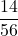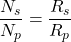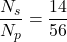The resistances of the primary and secondary coils of a transformer are 56 and 14 , respectively. Both coils are made from lengths of the s

Question

The resistances of the primary and secondary coils of a transformer are 56 and 14 , respectively. Both coils are made from lengths of the same copper wire. The circular turns of each coil have the same diameter. Find the turns ratio Ns /Np.

in progress 0
5 months 2021-08-06T09:32:36+00:00 1 Answers 21 views 0Explanation:

Given,

Resistance of the primary coil, R_p = 56 Ω

Resistance of the secondary coil, R_s = 14 Ω

We know,

Number of winding in the transformer is directly proportional to the length of the wire.

If the length of the wire increase the resistance also increase.

So, we can say that Number of turns is directly proportional to the resistance of the wire.

So,The turn ratio is equal to